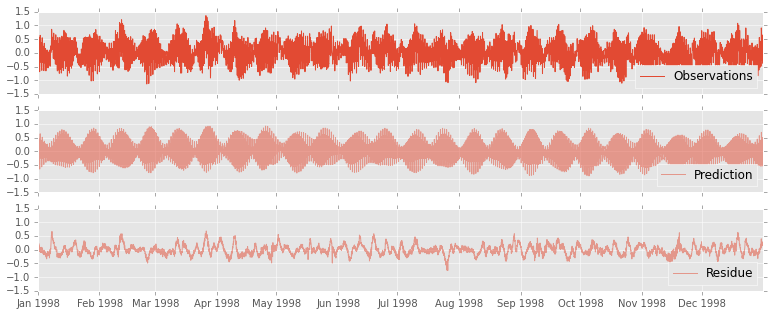# UTide for python

Right after I test ttide_py a friend told me about the python version of the Unified Tidal Analysis and Prediction (UTide) written by Wesley Bowman.

UTide seems to be a significant advance over t_tide. So let's try the python version! I will use the same data from my previous t_tide posts for comparison.

In :
from datetime import datetime

def date_parser(year, day, month, hour):
year, day, month, hour = map(int, (year, day, month, hour))
return datetime(year, day, month, hour)

parse_dates = dict(datetime=['year', 'month', 'day','hour'])

names = ['year', 'month', 'day', 'hour', 'elev', 'flag']

names=names,
skipinitialspace=True,
delim_whitespace=True,
index_col='datetime',
usecols=range(1, 7),
na_fvalues='9.990',
parse_dates=parse_dates,
date_parser=date_parser)

Out:
elev flag
datetime
1998-01-01 00:00:00 1.20 0
1998-01-01 01:00:00 1.43 0
1998-01-01 02:00:00 1.73 0
1998-01-01 03:00:00 2.03 0
1998-01-01 04:00:00 2.38 0
1998-01-01 05:00:00 2.54 0

Now let's remove the mean and interpolate the bad data points.

In :
import numpy as np
import matplotlib.pyplot as plt

corrected = obs['flag'] == 1

obs['anomaly'] = obs['elev'] - obs['elev'].mean()
obs['anomaly'] = obs['anomaly'].interpolate()


Python UTide is still in its early development stage. There are no docs at this point.

I opened an issue regarding the input/output description.

For now I am assuming it takes a matplotlib date2num data for the time argument. Note also that we have to specify all the inputs, even when they are empty.

In :
from utide import ut_solv
from matplotlib.dates import date2num

lat = -25.0147

time = date2num(obs.index.to_pydatetime())

coef = ut_solv(time, obs['anomaly'].values, np.array([]), lat, cnstit='auto',
notrend=True, rmin=0.95, method='ols',
nodiagn=True, linci=True, conf_int=True)

ut_solv:
matrix prep ...
Solution ...
conf. int vls...
Done.



Now let's reconstruct the tidal series,

In :
from utide import ut_reconstr

xout, _ = ut_reconstr(time, coef)

ut_reconstr:
prep/calcs...
Done.



and plot them.

In :
fig, (ax0, ax1, ax2) = plt.subplots(nrows=3, sharey=True, sharex=True, figsize=(13, 5))

ax0.plot(obs.index, obs['anomaly'], label=u'Observations')
ax0.legend(numpoints=1, loc='lower right')

ax1.plot(obs.index, xout, alpha=0.5, label=u'Prediction')
ax1.legend(numpoints=1, loc='lower right')

ax2.plot(obs.index, obs['anomaly']-xout, alpha=0.5, label=u'Residue')
_ = ax2.legend(numpoints=1, loc='lower right')The python version of UTide needs some help. Try it out, open issues, sent PRs, request features etc.

In :
HTML(html)

Out:

This post was written as an IPython notebook. It is available for download or as a static html.